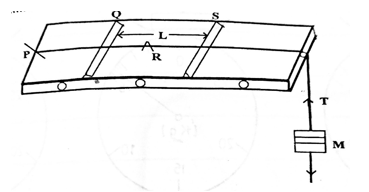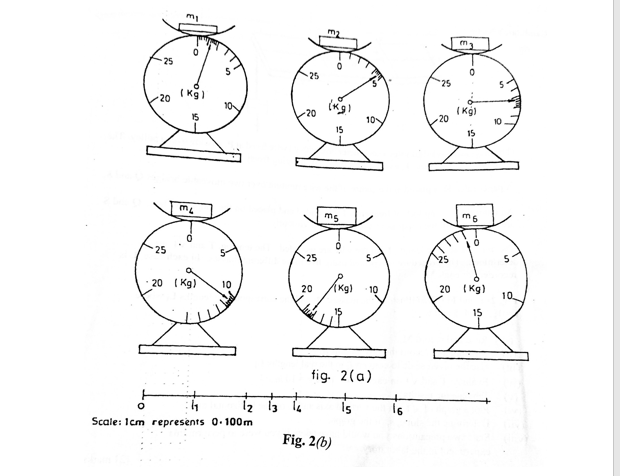Question 2The diagram above illustrates a sonometer box with its wire fixed at P, passing over a pulley.

The wire is under a tension, T, exerted by a mass, M, hanging freely at the end.

A paper rider, R is placed at the centre of the wire running over two moveable bridges Q and S are adjusted until the paper rider starts vibrating violently.

The mass M and the length, L, of the wire are recorded. The tension, T and √T are determined. The procedure is repeated using five more different masses. In each case, L is recorded for each M.
Fig 2(a) and Fig 2(b) illustrate the masses Mi and the corresponding lengths Li where i = 1. 2, 3, 4, 5, and 6.Read and record Mi.
Measure and record the raw length li

Use the given scale to convert li to the real lengths, Li

Evaluate T and √T in each case. Take g = 10 ms-2

Tabulate the results.

Plot a graph with √T on the vertical axis and L on the horizontal axis.

Determine the slope, s, of the graph

Evaluate.

State two precautions you would take if you would take if you were to perform this experiment in the laboratory.

i) From your graph, determine the value of f, if L, f, and T are related by
√T = fL/(15.1)
(ii) Explain the term resonance.

Observation

Part (a) Performance was below average. Moss candidates evaluated h-1 and y-1 of the raw values instead of the converted values.

Also, many candidates plotted the reciprocals of the raw values of h an y instead of converting them before finding the reciprocals.

Part (b) Candidates’ responded poorly in this part as many candidates could not differentiate a mirror from a lens and a convex mirror from a concave mirror.

The expected response is as stated below:

Six values of M/m correctly read and recorded to at least

1 d.p and within tolerance of ± 0.1kg

(Award ½ mark each)

Six values of l correctly measured and recorded to at

least 1 d.p. and within tolerance of ± 0.1 cm

(Award ½ mark each)

Six values of L correctly converted in metres

(Deduct ½ mark for each wrong or missing value)

Six values of T correctly evaluated.

(Deduct ½ mark for each wrong or missing value)

Six values of √T correctly evaluated to at least 3 s.f.

(Deduct ½ mark for each wrong or missing value)

Composite table showing at least M/m, l, L, T and √T.

Plot a graph using reasonable scales

draw line of best it

determine the slope of the graph

state any two of the following precautions.

e.g. - Avoid parallax error in metre rule.